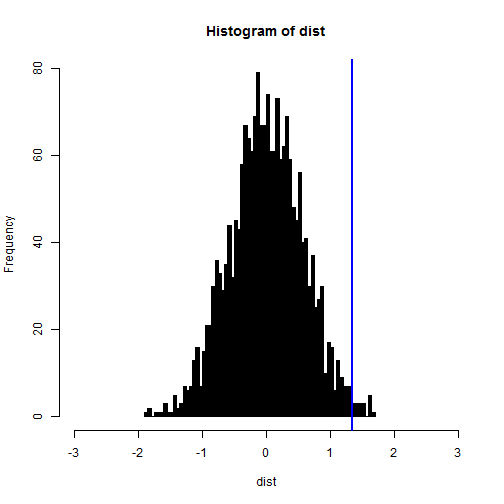# Permutation Tests

An increasingly common statistical tool for constructing sampling distributions is the permutation test (or sometimes called a randomization test). Like bootstrapping, a permutation test builds - rather than assumes - sampling distribution (called the “permutation distribution”) by resampling the observed data. Specifically, we can “shuffle” or permute the observed data (e.g., by assigning different outcome values to each observation from among the set of actually observed outcomes). Unlike bootstrapping, we do this without replacement.

Permutation tests are particularly relevant in experimental studies, where we are often interested in the sharp null hypothesis of no difference between treatment groups. In these situations, the permutation test perfectly represents our process of inference because our null hypothesis is that the two treatment groups do not differ on the outcome (i.e., that the outcome is observed independently of treatment assignment). When we permute the outcome values during the test, we therefore see all of the possible alternative treatment assignments we could have had and where the mean-difference in our observed data falls relative to all of the differences we could have seen if the outcome was independent of treatment assignment. While a permutation test requires that we see all possible permutations of the data (which can become quite large), we can easily conduct “approximate permutation tests” by simply conducting a vary large number of resamples. That process should, in expectation, approximate the permutation distribution.

For example, if we have only n=20 units in our study, the number of permutations is:

factorial(20)

##  2.433e+18


That number exceeds what we can reasonably compute. But we can randomly sample from that permutation distribution to obtain the approximate permutation distribution, simply by running a large number of resamples. Let's look at this as an example using some made up data:

set.seed(1)
n <- 100
tr <- rbinom(100, 1, 0.5)
y <- 1 + tr + rnorm(n, 0, 3)


The difference in means is, as we would expect (given we made it up), about 1:

diff(by(y, tr, mean))

##  1.341


To obtain a single permutation of the data, we simply resample without replacement and calculate the difference again:

s <- sample(tr, length(tr), FALSE)
diff(by(y, s, mean))

##  -0.2612


Here we use the permuted treatment vector s instead of tr to calculate the difference and find a very small difference. If we repeat this process a large number of times, we can build our approximate permutation distribution (i.e., the sampling distribution for the mean-difference). We'll use replicate do repeat our permutation process. The result will be a vector of the differences from each permutation (i.e., our distribution):

dist <- replicate(2000, diff(by(y, sample(tr, length(tr), FALSE), mean)))


We can look at our distribution using hist and draw a vertical line for our observed difference:

hist(dist, xlim = c(-3, 3), col = "black", breaks = 100)
abline(v = diff(by(y, tr, mean)), col = "blue", lwd = 2)At face value, it seems that our null hypothesis can probably be rejected. Our observed mean-difference appears to be quite extreme in terms of the distribution of possible mean-differences observable were the outcome independent of treatment assignment. But we can use the distribution to obtain a p-value for our mean-difference by counting how many permuted mean-differences are larger than the one we observed in our actual data. We can then divide this by the number of items in our permutation distribution (i.e., 2000 from our call to replicate, above):

sum(dist > diff(by(y, tr, mean)))/2000  # one-tailed test

##  0.009

sum(abs(dist) > abs(diff(by(y, tr, mean))))/2000  # two-tailed test

##  0.018


Using either the one-tailed test or the two-tailed test, our difference is unlikely to be due to chance variation observable in a world where the outcome is independent of treatment assignment.

## library(coin)

We don't always need to build our own permutation distributions (though it is good to know how to do it). R provides a package to conduct permutation tests called coin. We can compare our p-value (and associated inference) from above with the result from coin:

library(coin)
independence_test(y ~ tr, alternative = "greater")  # one-tailed

##
##  Asymptotic General Independence Test
##
## data:  y by tr
## Z = 2.315, p-value = 0.01029
## alternative hypothesis: greater

independence_test(y ~ tr)  # two-tailed

##
##  Asymptotic General Independence Test
##
## data:  y by tr
## Z = 2.315, p-value = 0.02059
## alternative hypothesis: two.sided


Clearly, our approximate permutation distribution provided the same inference and a nearly identical p-value. coin provides other permutation tests for different kinds of comparisons, as well. Almost anything that you can address in a parametric framework can also be done in a permutation framework (if substantively appropriate). and anything that coin doesn't provide, you can build by hand with the basic permutation logic of resampling.